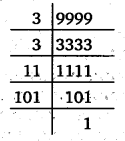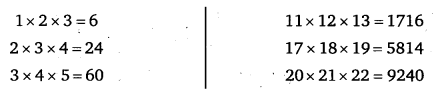# Class 6 Maths NCERT Solutions for Chapter 3 Playing with Numbers Ex 3.5

## Playing with Numbers

Question 1.
Which of the following statements are true?
(a)
If a number is divisible by 3, it must be divisible by 9.
(b) If a number is divisible by 9, it must be divisible by 3.
(c) A number is divisible by 18, if it is divisible by both 3 and 6.
(d) If a number is divisible by 9 and 10 both, then it must be , divisible by 90.
(e) If two numbers are co-primes’, at least one of them must be prime.
(f) All numbers which are divisible by 4 must also be divisible by 8.
(g) All numbers which are divisible by 8 must also be divisible by 4.
(h) If a number exactly divides two numbers separately, it must exactly divide their sum.
(i) If a number exactly divides the sum of two numbers, it must exactly divide the two numbers separately.

Solution:
Statements (b), (d), (g) and (i) are true.

Question 2.
Here are two different factor trees for 60. Write the missing numbers.Solution:Question 3.
Which factors are not included in the prime factorisation of a composite number?

Solution:
1 and composite numbers are not included in the prime factorisation of a composite number.

Question 4.
Write the greatest 4-digit number and express it in terms of its prime factors.

Solution:
The greatest 4-digit number = 9999
We have,∴ 9999 = 3 x 3 x 11 x 101

Question 5.
Write the smallest 5-digit number and express it in the form of its prime factors.

Solution:
Smallest 5-digit number = 10000
We have,∴ 10000=2 x 2 x 2 x 2 x 5 x 5 x 5 x 5

Question 6.
Find all the prime factors of 1729 and arrange them in ascending order. Now state the relation, if any; between two consecutive prime factors.

Solution:
We have,∴ 1729 = 7 x 13 x 19
Relation between two consecutive prime factors is stated as “difference between two consecutive prime factors is 6”.

Question 7.
The product of three consecutive numbers is always divisible by 6. Verify this statement with the help of some examples.

Solution:
Let us consider the product of three consecutive numbers as under :In all the products, we have 6 or 4 or 0 in the unit’s place, so each product is divisible by 2.
Also, sum of digits in these products are divisible by 3. So, each of the product is divisible by 3.
Since 2 and 3 are co-prime, so the product 2 x 3 = 6 divides each of the above products.
Thus, the product of three consecutive numbers is always divisible by 6.

Question 8.
The sum of two consecutive odd numbers is divisible by 4. Verify this statement with the help of same examples.

Solution:
3 + 5 = 8 and 8 is divisible by 4.
5 + 7 = 12 and 12 is divisible by 4.
7 + 9=16 and 16 is divisible by 4.
9 +11 = 20 and 20 is divisible by 4.

Question 9.
In which of the following expressions, prime factorisation has been done ? ‘
(a)
24 = 2 x 3 x 4
(b) 56 = 7 x 2 x 2 x 2
(c) 70 = 2 x 5 x 7
(d) 54 = 2 x 3 x 9

Solution:
In (b) and (c) prime factorisation has been done.

Question 10.
Determine if 25110 is divisible by 45.

Solution:
Since 45 = 5 x 9, where 5 and 9 are co-primes.
So to check the divisibility of 25110 by 45, test it for 5 and 9.
In 25110, unit’s digit = 0
So, 25110 is divisible by 5.
Sum of its digits = 2 + 5 + 1 + 1+ 0 = 9, which is divisible by 9.
So, 25110 is also divisible by 9.
Hence, 25110 is divisible by 45.

Question 11.
18 is divisible by both 2 and 3. It is also divisible by 2 x 3 = 6. Similarly, a number is divisible by both 4 and 6. Can we say that the number must also be divisible by 4 x 6 = 24 ? If not, give an example to justify your answer.

Solution:
Not necessarily, as the numbers 12,36,60 etc. are each divisible by both 4 and 6. But these numbers are not divisible by 4 x 6 = 24.

Question 12.
I am the smallest number, having four different prime factors. Can you find me?

Solution:
Smallest four different prime numbers are 2, 3, 5 and 7.
∴ Required number = 2 x 3 x 5 x 7 = 210.